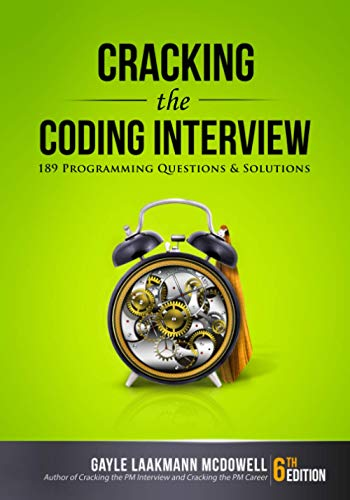# Reverse the Order of Words in a String

Question: Reverse the order of words in a string in C using as little additional memory as possible.

Answer: The solution for this questions uses the solution of Reverse a String. I am going to make a small change to that solution by adding a length parameter. It will probably be better for you to read through that question before proceeding further with this one. Note that a space is being used to delimit the words in the sentence. Let’s look at an example before we dive into the code.

Original Sentence: My car is very fast

Modifies Sentence: fast very is car My

```void reverseWords( char * str )
{
int i = 0, j = 0;
reverseString( str, strlen(str) ); // tsaf yrev si rac yM
while( 1 ) // Loop forever
{
if( *(str+j) == ' ' || *(str+j) == '\0') // Found a word or reached the end of sentence
{
reverseString( str+i, j-i );
i = j+1;
}
if( *(str+j) == '\0')
{
break;
}
j++;
}
}```
```void reverseString(char* str, int len)
{
int i, j;
char temp;
i=j=temp=0;

j=len-1;
for (i=0; i<j; i++, j--)
{
temp=str[i];
str[i]=str[j];
str[j]=temp;
}
}```

I did not have time to test this a lot so if there are any mistakes, please let me know.

If you have any questions, please feel free to send me an email at [email protected]. If you have any interview questions which you feel would benefit others, I would love to hear about it.

If you're looking for some serious preparation for your interviews, I'd recommend this book written by a lead Google interviewer. It has 189 programming questions and solutions:## 5 Responses

1.Jitendra says:

for (i=0; i<j; i++, j–)
{
temp=str[i];
str[i]=str[j];
str[j]=temp;
}

Here you should check for i<j/2

2.rask says:

Little modification – just using one counter and a more elegant length/2:

void reverseString(char* str)
{
puts(str);
int i=0;
char temp;
int length=strlen(str);
for (i=0; i<length/2; i++){
temp=str[i];
printf("CHAR IS %c\n", str[i]);
str[i] = str[length-i-1];
str[length-i-1] = temp;
}
}

3.mhassan83 says:

``` static String reverseString(String str) { char[] strArray = str.toCharArray(); int j = strArray.length - 1; for (int i = 0; i < j; i++, j--) { strArray[i] ^= strArray[j]; strArray[j] ^= strArray[i]; strArray[i] ^= strArray[j]; }```

``` return new String(strArray); } static String reverseLine(String line) { String reverseString = reverseString(line); StringBuffer temp = new StringBuffer(); StringBuffer result = new StringBuffer(); for (int i =0; i 0) { result.append(reverseString(temp.toString())); temp.delete(0, temp.length()); } result.append(reverseString.charAt(i)); } else { temp.append(reverseString.charAt(i)); } } // appends the last string if any if (temp.length() > 0) { result.append(reverseString(temp.toString())); } ```

``` return result.toString(); } ```

4.Raj says:

nice one…….

5.Reinhardt says:

danke!

XHTML: These are some of the tags you can use: `<a href=""> <b> <blockquote> <code> <em> <i> <strike> <strong>`